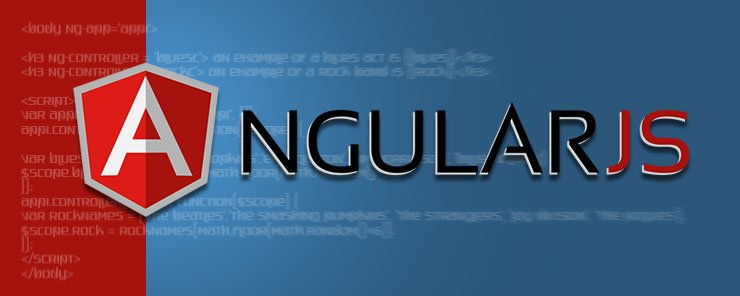Web Programming TutorialsLearn about The Scope Object in AngularJS

# Learn about The Scope Object in AngularJSAs we’ve seen in the AngularJS controller tutorial, the scope component is used to provide data to the view. In other words, it links HTML elements to variables inside the factory function of the controller (Javascript data), and is available to both sides of the MVC model . Changes in the view result in changes to the controller and thus values of the scope properties/methods.
The scope object is always passed as a parameter in the controller set up function; By writing function(\$scope) we’re telling AngularJS to pass the scope object on to the function whenever it is called.

For our example in this AngularJS tutorial, we’ll demonstrate the use of multiple controllers (each using a scope object) for the same Angular application. Lets start with the HTML and reference a new application app1 and two different controllers, like so:

`<body ng-app='app1'>`
```<h3 ng-controller = 'BluesC'> An example of a blues act is {{blues}}</h3>
<h3 ng-controller = 'RockC'> An example of a rock band is {{rock}}</h3>```

We have two expressions containing two \$scope properties. Let’s create the Javascript:

```<script>
var app1 = angular.module('app1', []);
app1.controller('BluesC', function(\$scope) {

var bluesNames = ['Lightnin Hopkins','Eric Clapton', 'Jim Morrisson', 'Billie Holiday'];
\$scope.blues = bluesNames[Math.floor(Math.random()*5)];
});
app1.controller('RockC', function(\$scope) {
var rockNames = ['The Beatles','The Smashing Pumpkins', 'The Stranglers', 'Joy Division', 'The Pogues'];
\$scope.rock = rockNames[Math.floor(Math.random()*6)];
});
</script>
</body>
```

In the script, we defined app1 and set up two controllers for it, separately. The controller named BluesC has a variable containing an array of strings (‘bluesNames’) and a scope object with a property of ‘blues’.

The controller named RockC has a variable containing an array of strings (‘rockNames’) and a scope object with a property of ‘rock’.

The Math.random() method returns a random number from 0 (inclusive) and up to but not including 1. Math.floor is a method which rounds a number downward to its nearest integer. Therefore, Math.floor((Math.random() * 10) + 1), for example, would yield a random number between 1 and 10. We can use the Math.floor and Math.random() methods, together, to pick a random value from an array. The general formula would then be:

`ArrayName[Math.floor((Math.random()*(ArrayName.length))];`

As we’ve seen in the example above, the view (HTML) can access any property that we add to the scope object. In the HTML, we only indicate the propertyname(in this case, ‘blues’ or ‘rock’), and not the \$scope prefix, to summon the value of the property.Here’s another simple example, where you can see the scope object “in action”:

The view/HTML:

```<body ng-app='app1'>
<h3 ng-controller = 'Grocery'> We need to get{{groc.length}} different things from the supermarket</h3>
```

Let’s create the JavaScript:

```<script>
var app1 = angular.module('app1', []);
app1.controller('Grocery', function(\$scope) {
\$scope.groc = ['banana', 'apple','milk']
});
</script>
</body>
```

The result would be the length of the groc array displayed to the user in place of the first expression.

In summary, we can think of the AngularJS application as composed of 3 elements – the view/HTML, the controller function which manages the data “backstage”, and the scope which is the model/the data itself that’s accessible to both the HTML and the “backstage”. The latter essentially connects the two.

### Why Is Design Thinking Important In Digital Transformation?

21,501Fans
4,210Followers
104,000Subscribers

### Why Is Design Thinking Important In Digital Transformation?

- Advertisement -